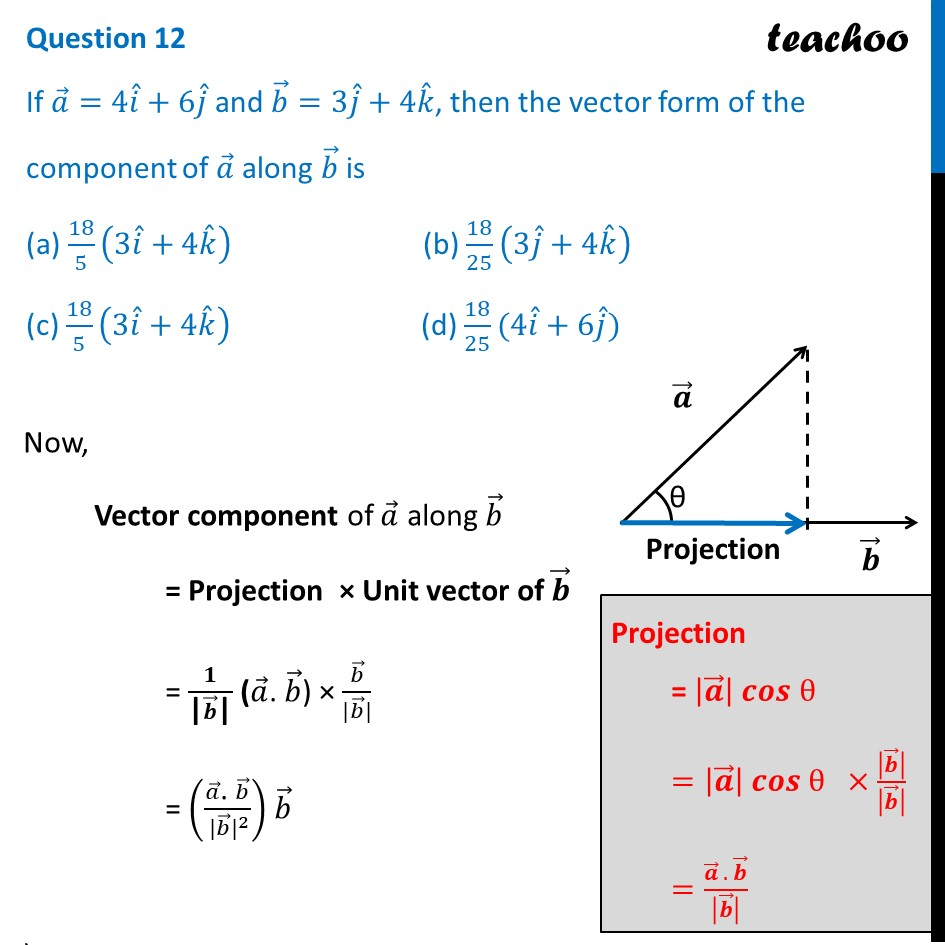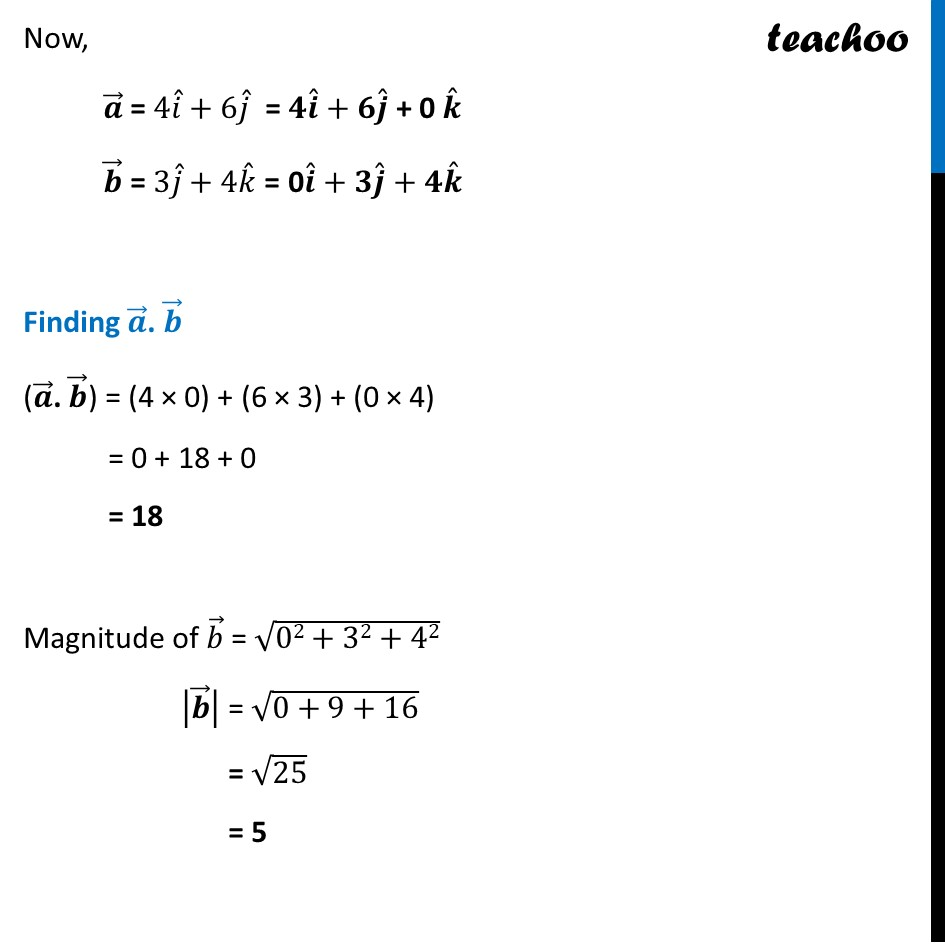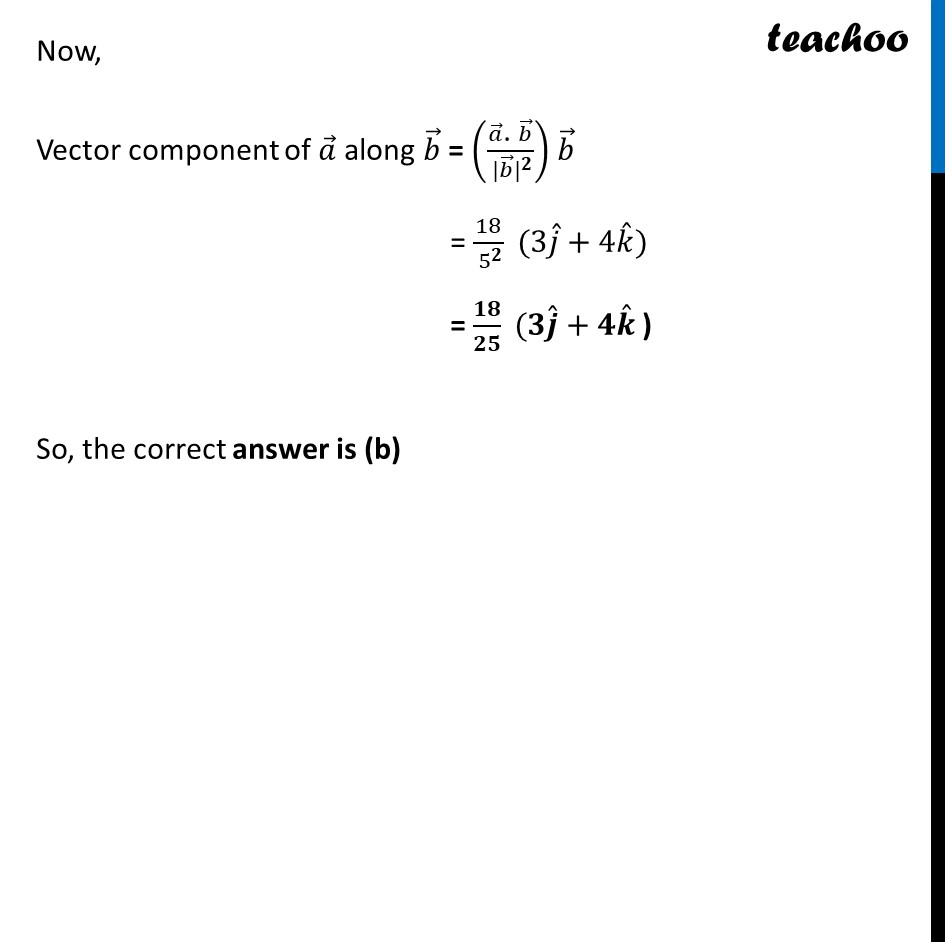CBSE Class 12 Sample Paper for 2024 Boards

Class 12
Solutions of Sample Papers and Past Year Papers - for Class 12 Boards

## (c) 18/5 (3i ˆ+4k ˆ )                            (d) 18/25(4i ˆ+6j ˆ)Learn in your speed, with individual attention - Teachoo Maths 1-on-1 Class

### Transcript

Now, Vector component of 𝑎 ⃗ along 𝑏 ⃗ = Projection × Unit vector of 𝒃 ⃗ = 𝟏/("|" 𝒃 ⃗"|" ) (𝑎 ⃗. 𝑏 ⃗) × 𝑏 ⃗/(|𝑏 ⃗|) = ((𝑎 ⃗". " 𝑏 ⃗)/〖\ |𝑏 ⃗|〗^2 ) 𝑏 ⃗ ) Now, 𝒂 ⃗ = 4𝑖 ˆ+6𝑗 ˆ = 𝟒𝒊 ˆ+𝟔𝒋 ˆ + 0 𝒌 ˆ 𝒃 ⃗ = 3𝑗 ˆ+4𝑘 ˆ = 0𝒊 ˆ + 𝟑𝒋 ˆ+𝟒𝒌 ˆ Finding 𝒂 ⃗. 𝒃 ⃗ (𝒂 ⃗. 𝒃 ⃗) = (4 × 0) + (6 × 3) + (0 × 4) = 0 + 18 + 0 = 18 Magnitude of 𝑏 ⃗ = √(02+32+42) |𝒃 ⃗ | = √(0+9+16) = √25 = 5 Now, Vector component of 𝑎 ⃗ along 𝑏 ⃗ = ((𝑎 ⃗". " 𝑏 ⃗)/〖\ |𝑏 ⃗|〗^𝟐 ) 𝑏 ⃗ = 18/〖\ 5〗^𝟐 (3𝑗 ˆ+4𝑘 ˆ) = 𝟏𝟖/𝟐𝟓 (𝟑𝒋 ˆ+𝟒𝒌 ˆ ) So, the correct answer is (b)